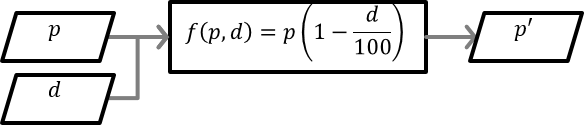# Rudiments of Linear Algebra & Computer Graphics

Author: Pirouz Nourian Assistant Professor of Design Informatics at TU Delft, Faculty of Architecture and Built Environment, Department of Architectural Engineering Technology,
Last edited in August 2019

Note: This is still a work in progress and there are some errors in the visualization and rendering of the mathematical notations

## Introduction

This is a very short introduction for impatient Computer Graphics and Computational Design practitioners. Linear Algebra is a branch of mathematics. It is called linear algebra because it is the study of linear operators in an algebraic way (as opposed to, say, an arithmetic approach). Linearity is somewhat more twisted that you might think so we first explain what is meant by algebra.

## Linear Algebra

### Algebra

Suppose you are shopping for an item of clothing worth 14.99 bucks and that there is a discount percentage of 17.5 percent on it. To calculate the discount price you would find the 17.5 percent of 14.99 and subtract it from 14.99. This would be an arithmetic approach. Instead, if you generalize the problem and transcend the numbers to numerical variables (a.k.a. parameters or arguments), you can write: $$\begin{equation} p'= p(1-\frac{1}{100}) \end{equation}$$ This formula is now working like a machine (a function) that maps the price of every item dubbed p with a discount percentage d, to a new discount price denoted as p'. Conceptually, this machince can be concieved as a black box with inputs and outputs (possibly more than one):a function conceptualized as a machine,,schematized in the visual language of flowcharts, practically, this can be a Python function.A function of some scalar variables in a set to a variable in some other set is often figuratively described as a map and is shown as $f:v↦w$ (read as function f that maps v to w), where the set v is referred to as the [input] domain and the set w is referred to as the [output] range (a.k.a. codomain) of the function in question.

def DiscountPrice(p,d):
p_prime=p*(1-d/100)
return p_prime


The keyword ‘def’ in Python marks a function definition. The keyword ‘return’ brings the imaginary cursor/calculator where it used to be, i.e. before calling th function and thus it effectively delivers the output[s] of the function.

### Dimensionality

It might appear from the above definition that functions can only put out one variable at a time. However, we can easily generalize functions to take in and put out more than a single number. That is the essential reason to go to higher dimensions and define vectors, matices, and tensors. Although a geometric intuition (1D, 2D, and 3D) is often helpful to visualize multi-dimensionality, it can also be misleading. Dimensionality is a concept that has to do with combinations of (often numerical) elements from sets. $$\begin{equation} \begin{cases} & \mathbb{R}^1=\mathbb{R} \text{ one dimensional vectors or scalars }\\ & \mathbb{R}^2=\mathbb{R}\times \mathbb{R}:={(x,y)|x\in \mathbb{R} \wedge y\in \mathbb{R}} \\ & \mathbb{R}^3=\mathbb{R}\times \mathbb{R}\times \mathbb{R} :={(x,y,z)|x\in \mathbb{R} \wedge y\in \mathbb{R} \wedge z\in \mathbb{R}} \\ \end{cases} \end{equation}$$